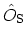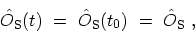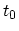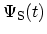# B.1 SCHRÖDINGER Picture

In the SCHRÖDINGER picture the operatorsare time-independent(B.2)

whereis assumed to be the time reference point. The time dependence of the state vectoris obtained from the SCHRÖDINGER equation(B.3)

which has the formal solution(B.4)

M. Pourfath: Numerical Study of Quantum Transport in Carbon Nanotube-Based Transistors